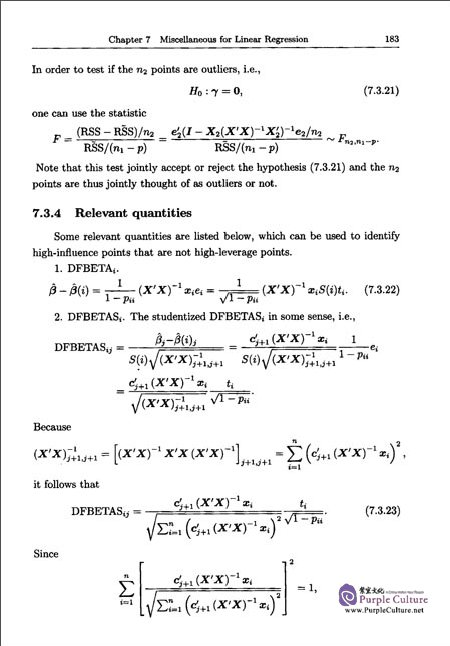FEEDBACK

#### Regression Analysis

Price: \$11.19 \$7.86 (Save \$3.33)

Author: WU Ping; Wu Xianyi;
Language: English
Format: 22.4 x 16.6 x 2 cm
Page: 215
Publication Date: 08/2016
ISBN: 9787302446309
Publisher: Tsinghua University Press
Chapter 1 Preliminaries： Matrix Algebra and Random Vectors
1.1 Preliminary matrix algebra
1.1.1 Trace and eigenvalues
1.1.2 Symmetric matrices
1.1.3 Idempotent matrices and orthogonal projection
1.1.4 Singular value decomposition
1.1.5 Vector differentiation and generalized inverse
1.1.6 Exercises
1.2 Expectation and covariance
1.2.1 Basic properties
1.2.2 Mean and variance of quadratic forms
1.2.3 Exercises
1.3 Moment generating functions and independence
1.3.1 Exercises
Chapter 2 Multivariate Normal Distributions
2.1 Definitions and fundamental results
2.3 Exercises
Chapter 3 Linear Regression Models
3.1 Introduction
3.2 Regression interpreted as conditional mean
3.3 Linear regression interpreted as linear prediction
3.4 Some nonlinear regressions
3.5 Typical data structure of linear regression models
3.6 Exercises
Chapter 4 Estimation and Distribution Theory
4.1 Least squares estimation （LSE）
4.1.1 Motivation： why is LS reasonable
4.1.2 The LS solution
4.1.3 Exercises
4.2 Properties of LSE
4.2.1 Small sample distribution—free properties
4.2.2 Properties under normally distributed errors
4.2.3 Asymptotic properties
4.2.4 Exercises
4.3 Estimation under linear restrictions
4.4 Introducing further explanatory variables and related topics
4.4.1 Introducing further explanatory variables
4.4.2 Centering and scaling the explanatory variables
4.4.3 Estimation in terms of linear prediction
4.4.4 Exercises
4.5 Design matrices of less than full rank
4.5.1 An example
4.5.2 Estimability
4.5.3 Identifiability under Constraints
4.5.4 Dropping variables to change the model
4.5.5 Exercises
4.6 Generalized least squares
4.6.1 Basic theory
4.6.2 Incorrect specification of variance matrix
4.6.3 Exercises
4.7 Bayesian estimation
4.7.1 The basic idea
4.7.2 Normal—noninformative structure
4.7.3 Conjugate priors
4.8 Numerical examples
4.9 Exercises
Chapter 5 Testing Linear Hypotheses
5.1 Linear hypotheses
5.2 F—Test
5.2.1 F—test
5.2.2 What are actually tested
5.2.3 Examples
5.3 Confidence ellipse
5.4 Prediction and calibration
5.5 Multiple correlation coefficient
5.5.1 Variable selection
5.5.2 Multiple correlation coefficient： straight line
5.5.3 Multiple correlation coefficient： multiple regression
5.5.4 Partial correlation coefficient
5.6 Testing linearity： goodness—of—fit test
5.7 Multiple comparisons
5.7.1 Simultaneous inference
5.7.2 Some classical methods for simultaneous intervals
5.8 Univariate analysis of variance
5.8.1 ANOVA model
5.8.2 ANCOVA model
5.8.3 SAS procedures for ANOVA
5.9 Exercises
Chapter 6 Variable Selection
6.1 Impact of variable selection
6.2 Mallows' Cp
6.3 Akaike's information criterion （AIC）
6.3.1 Prelimilaries： asymptotic normality of MLE
6.3.2 Kullback—Leibler's distance
6.3.3 Akaike's Information Criterion
6.3.4 AIC for linear regression
6.4 Bayesian information criterion （BIC）
6.5 Stepwise variable selection procedures
6.6 Some newly proposed methods
6.6.2 Nonnegative garrote
6.7 Final remarks on variable selection
6.8 Exercises
Chapter 7 Miscellaneous for Linear Regression
7.1 Collinearity
7.1.1 Introduction
7.1.2 Examine collinearity
7.1.3 Remedies
*7.2 Some remedies for collinearity
7.2.1 Ridge regression
7.2.2 Principal Component Regression
7.2.3 Partial least square
7.2.4 Exercises
7.3 Outliers
7.3.1 Introduction
7.3.2 Single outlier
7.3.3 Multiple outliers
7.3.4 Relevant quantities
7.3.5 Remarks
7.4 Testing features of errors
7.4.1 Serial correlation and Durbin—Watson test
7.4.2 Testing heteroskeasticity and related topics
7.5 Some extensions and variants
7.5.1 Box—Cox model
7.5.2 Modeling the variances
7.5.3 A remark
Chapter 8 Logistic Regression： Modeling Categorical Responses
8.1 Logistic regression
8.1.1 Logistic regression for dichotomous responses
8.1.2 Likelihood function for logistic regression
8.1.3 Interpreting the logistic regression
8.2 Multiple logistic regression
8.2.1 Maximum likelihood estimation for multiple logistic regression
8.3 Inference for logistic regression
8.3.1 Inference for simple logistic regression
8.3.2 Inference for multiple logistic regression
8.4 Logistic regression for multinomial responses
8.4.1 Nominal responses baseline—category logistic regression
8.4.2 Ordinal responses： cumulative logistic regression
8.5 Exercises
Bibliography
##### Sample Pages Preview\$7.86